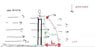# How to solve this problem?

• sittisakbj

#### sittisakbj

Hello there,
how i can solve and what 's answer for this problem(L=?) ?

#### Attachments

•me.jpg
16.3 KB · Views: 489
You need to post the original question...

sorry
i have not original question because some one ask me for this question and i sketch this image by my self .
problem is: i hold mass 1 kg then i liberate it at height 50 m .at this time have the wind blows in horizontal from the left goes to right 20km/hr . if g = 9.81m/s^ 2 . How much is this mass fall far from original point ( horizontal distance )?

the first place to start is how long it takes for the ball to hit the ground.
this should be very straight forward.

then use that amount of time to see how far the ball travels in the x direction in this amount of time. hint: you are given the velocity in the x direction.

Hope this helps!